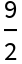# TotalVariation

Contributed by: Wolfram Research

Get the total variation of a matrix

 ResourceFunction["TotalVariation"][matrix] gives the total variation for matrix.

## Details and Options

ResourceFunction["TotalVariation"][matrix] effectively gives the trace of the covariance matrix for matrix.
ResourceFunction["TotalVariation"][matrix] is equivalent to Total[Variance[matrix]].

## Examples

### Basic Examples

Total variation of bivariate data:

 In:=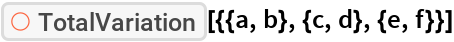Out=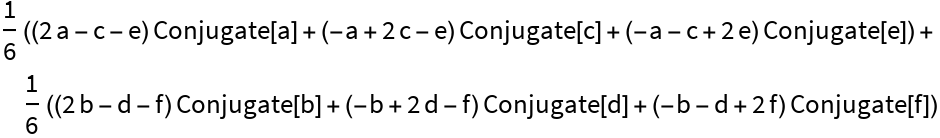Assume all symbols are real and simplify:

 In:=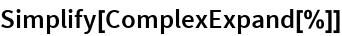Out=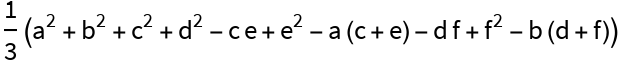### Properties & Relations

Total variation is equivalent to the trace of the covariance matrix:

 In:=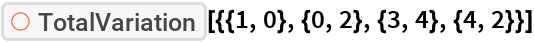Out=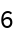In:=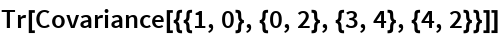Out=Total variation is equivalent to the sum of column variances:

 In:=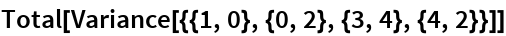Out=### Options

Use the maximum likelihood estimation:

 In:=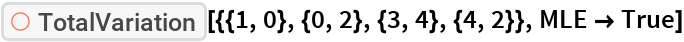Out=